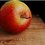# Rubik's Cube ProofIn this note, we will proof that repeatedly applying any algorithm on a solved Rubik's Cube would cause it to eventually return back to its solved state.

In other words, supposed the algorithm is to turn the top side once. After repeating this algorithm 4 times, the cube would be returned to its solved state. In the problem above, “any algorithm” would refer to literally any algorithm. For instance, your algorithm might be: R’ D D R D R’ D’ R, and repeating this algorithm a sufficient number of times on a solved cube would make it eventually return to its solved state.

This problem seems tough. However, the solution to this problem requires no prerequisites, just a grasp of logic.

Solution:

To tackle this problem, I will be using this technique called Proof by Contradiction

For instance, if I want to proof that statement A is false, I assume otherwise; that A is true. Then I show that if A is true, absurd stuff that doesn’t make sense would happen (ie. we would see a contradiction). Since A being true causes a contradiction, A must be false. Hence proved that A is false. It works the other way too; proving A is true.

Let’s first assume that there exists an algorithm (Let’s call it $f$) that would not cycle. In other words, repeatedly applying $f$ on a cube would cause it to always land in a state that it has not been before:However, this would imply that a rubik's cube has an INFINITE number of different states! This is a contradiction, since it is well known that a rubik's cube has a finite number of states (Left as exercise of reader). Since there is a contradiction, we can safely conclude that there exists no such $f$.

The above conclusion results in 2 possible cases:Where the cube eventually returns to the solve state (which we want)Where the cube returns not to the solved state but to some random state within the cycle (which we want to disprove).

To disprove Case 2, Proof by Contradiction can be applied once again. Let $f^{-1}$ be the inverse algorithm of $f$. Basically, $f^{-1}$ reverses what $f$ does to the cube:Now we replace all $f$ with $f^{-1}$ in Case 2.Focus on "State n". Notice how this diagram implies that applying the algorithm “f^{-1}” on state n would result in 2 possible states (State n-1 and State k). This is absurd because we know that applying an algorithm on a rubik's cube will result in only 1 possible state (ie. each state should only point to 1 other state). Hence we have reached a conclusion that Case 2 is IMPOSSIBLE. Which leaves us with Case 1 as the only case that is possible.

Hence, proved, that repeatedly applying any algorithm on a solved Rubixs Cube would cause it to eventually return back to its solved state.Note by Julian Poon
3 years, 7 months ago

This discussion board is a place to discuss our Daily Challenges and the math and science related to those challenges. Explanations are more than just a solution — they should explain the steps and thinking strategies that you used to obtain the solution. Comments should further the discussion of math and science.

When posting on Brilliant:

• Use the emojis to react to an explanation, whether you're congratulating a job well done , or just really confused .
• Ask specific questions about the challenge or the steps in somebody's explanation. Well-posed questions can add a lot to the discussion, but posting "I don't understand!" doesn't help anyone.
• Try to contribute something new to the discussion, whether it is an extension, generalization or other idea related to the challenge.

MarkdownAppears as
*italics* or _italics_ italics
**bold** or __bold__ bold
- bulleted- list
• bulleted
• list
1. numbered2. list
1. numbered
2. list
Note: you must add a full line of space before and after lists for them to show up correctly
paragraph 1paragraph 2

paragraph 1

paragraph 2

[example link](https://brilliant.org)example link
> This is a quote
This is a quote
    # I indented these lines
# 4 spaces, and now they show
# up as a code block.

print "hello world"
# I indented these lines
# 4 spaces, and now they show
# up as a code block.

print "hello world"
MathAppears as
Remember to wrap math in $$ ... $$ or $ ... $ to ensure proper formatting.
2 \times 3 $2 \times 3$
2^{34} $2^{34}$
a_{i-1} $a_{i-1}$
\frac{2}{3} $\frac{2}{3}$
\sqrt{2} $\sqrt{2}$
\sum_{i=1}^3 $\sum_{i=1}^3$
\sin \theta $\sin \theta$
\boxed{123} $\boxed{123}$

Sort by:

In group theory, the group generated by repeated application of an action is called the cyclic group of that element. The number of times one has to apply an action to itself to get back the identity is called the order of the element. This can have interesting applications.

Here is a fun problem on the Rubik's Cube asking you to compute the order of certain elements of the Rubik's Group,

- 3 years, 7 months ago

Nice! Thanks for that information!

- 3 years, 7 months ago

This reminds me of the cyclic arguments like showing why the Fibonacci numbers mod n is immediately periodic. I wonder if there is a connection.

- 3 years, 7 months ago

There is. The same proof technique works.

- 3 years, 7 months ago

See YouTube videos on how to solve they will guide you. Don't apply your brain or it will be next to impossible.

- 3 years, 5 months ago